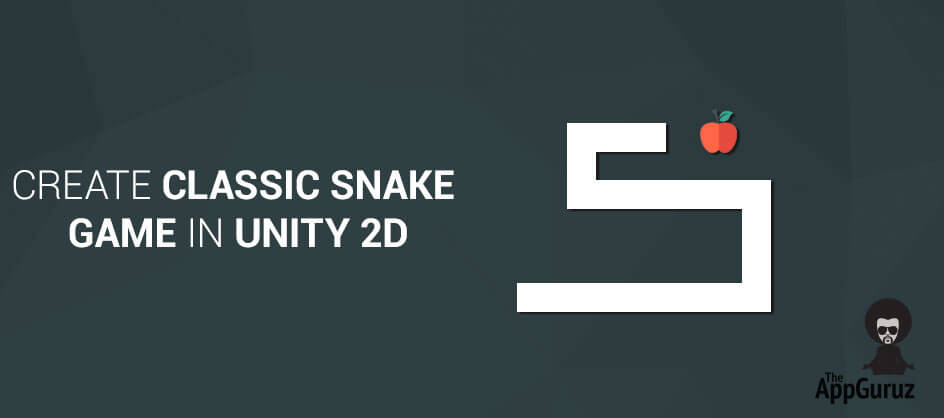## Objective

Main objective of this blog post is to give you an idea about how to Create Classic Snake Game in Unity 2D.

## Step 1 Detect Touch on First Object

Begin detecting the first object of the chain on touch. There are different logics for detecting objects out of which I have explained about circle overlapping.

``````Vector3cameraPosition = Camera.main.ScreenToWorldPoint(Input.mousePosition);
Collider2D[] others = Physics2D.OverlapCircleAll(cameraPosition, 1);
foreach(Collider2D collider in others)
{
if(collider.transform.CompareTag("First"))
{
//Object detected
isMoving = true;
break;
}
}

Update()
{
If(isMoving)
Move();
}
void Move()
{
Vector3nextPartPosition = transform.position;
QuaternionnextPartRotation = transform.localRotation;

currentPosition = transform.position;
Vector3mousePos = Input.mousePosition;

currentTouch = Camera.main.ScreenToWorldPoint(mousePos);
moveDirection = Utility.GetDirection(currentTouch, currentPosition);

// Find next position at designate distance between 2 object
Vector3nextPosition = currentPosition+(moveDirection * distanceBetweenTwoPart);

transform.position= Vector2.Lerp(transform.position, currentPosition, Time.deltaTime * Constants.SPEED);

if (moveDirection != Vector3.zero)
{
floattargetAngle = GetAngle(moveDirection);
transform.rotation = Quaternion.Slerp (transform.rotation, Quaternion.Euler (0, 0, targetAngle), 30f * Time.deltaTime);
}
// pass position & rotation to next object
snakePart.MoveSnakePart(nextPartPosition, nextPartRotation);

// if distance between object & touch position is greater than
// designate distance than call Move() method again
if(Vector2.Distance (transform.position, currentTouch) >distanceBetweenTwoPart)
{
Move();
}
}``````

Here, I got an array of all the objects in range of radius 1 at touch position using Physics2D.OverlapCircleAll() function. Chain will move when the first object is detected from those objects array.

If the first object is detected then step-2 to step-6 are executed in Update(). Continue until touch is not ended.

Chain movement stops when touch is ended.

## Step 2 Find Direction

Based on the new touch position find direction in which your chain should be moved.

``````public static Vector3 GetDirection(Vector3 firstPoint, Vector3 secondPoint)
{
Vector3 theRetDirection = Vector3.zero;
theRetDirection = firstPoint - secondPoint;
theRetDirection.z = 0;
theRetDirection.Normalize();
return theRetDirection;
}``````

Here, firstPoint is current touch position and secondPoint is object’s current position theRetDirection is normalized direction.

When normalized, a vector keeps the same direction but its length is 1.0.

## Step 3 Find Next Position and Move

According to the direction find next position for moving object

``````Vector3 nextPosition = transform.position + ( moveDirection * distanceBetweenTwoObject);
transform.position = Vector2.Lerp(transform.position, nextPosition, Time.deltaTime * Constants.SPEED);``````

Here, calculate the next position or target position. moveDirection is normalized direction, distanceBetweenTwoObject is floating point value which contains distance between two objects.

Using Lerp we can smoothly move objects form one point to another using designated speed & designated distance.

## Step 4 Find Angle

If we need to rotate an object then we have to find the angle. Below shown is the calculation of angle.

``````publicstaticfloatGetAngle(Vector3moveDirection)
{
floattargetAngle = Mathf.Atan2(moveDirection.y, moveDirection.x) * Mathf.Rad2Deg - 90;
returntargetAngle;
}``````

Here, moveDirection is normalized Direction. To calculate the angle we should turn towards, we deduct 90, it makes the sprite rotate.

## Step 5 Set Rotation

After getting the angle, we need to set the rotation of object using the following code.

``transform.rotation = Quaternion.Slerp (transform.rotation, Quaternion.Euler (0, 0, targetAngle), 30f * Time.deltaTime);``

Here, rotate the sprite or object using Slerp (from its previous rotation, to the new one) at the designated speed.

## Step 6 Set current position & rotation to next object

If we move the first object we have to move the whole chain. So, now second object should be moved on first object position, third object should be moved on second object position, forth object should be moved on third object position and so on.

So, here before we move the second object, we need to store the first object position.

``````Vector3nextObjectPosition = transform.position;
QuaternionnextObjectRotation = transform.localRotation;``````

Here, I’ve also stored rotation of first object because when second object moves on first object position then there may be changes in rotation.

``````publicvoidMoveOtherObject(Vector3nextPosition, QuaternionnextRotation)
{
Vector3nextPartPosition = transform.position;

QuaternionnextPartRotation = transform.localRotation;

transform.position= Vector2.Lerp(transform.position, nextPosition, Time.deltaTime * Constants.SPEED);

transform.rotation = Quaternion.Slerp (transform.rotation, nextRotation, 20f * Time.deltaTime);
snakePart.MoveSnakePart(nextPartPosition, nextPartRotation);
}``````

Here, MoveOtherObjectmethod applies for all objects other then the first one. Each object moves on previous position and makes place for the next object. This way the whole chain moves on touch.

I hope this blog post will help you to Create Classic Snake Game in Unity2D. Let me know in comment if you have any questions regarding Unity2D. I will reply you ASAP.

Got an Idea of Game Development? What are you still waiting for? Contact us now and see the Idea live soon. Our company has been named as one of the best Game Development Company in India.I am ambitious 2D Game developer with a Determined of learning new stuff and creating a optimistic future in game industry.